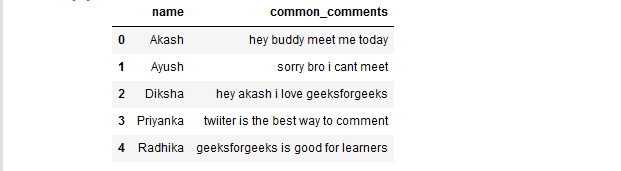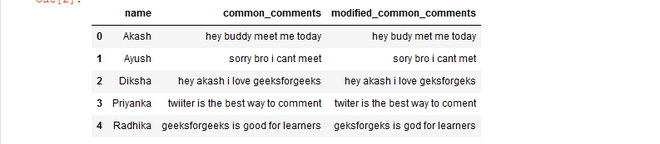# How to Remove repetitive characters from words of the given Pandas DataFrame using Regex?

Prerequisite: Regular Expression in Python

In this article, we will see how to remove continuously repeating characters from the words of the given column of the given Pandas Dataframe using Regex.

Here, we are actually looking for continuously occurring repetitively coming characters for that we have created a pattern that contains this regular expression (\w)\1+ here \w is for character, 1+ is for the characters that come more than once.

We are passing our pattern in the re.sub() function of re library.

Syntax: re.sub(pattern, repl, string, count=0, flags=0)

The ‘sub’ in the function stands for SubString, a certain regular expression pattern is searched in the given string(3rd parameter), and upon finding the substring pattern is replaced by repl(2nd parameter), count checks and maintains the number of times this occurs.

Now, Let’s create a Dataframe:

## Python3

 `# importing required libraries` `import` `pandas as pd` `import` `re`   `# creating Dataframe with column ` `# as name and common_comments` `df ``=` `pd.DataFrame(` `  ``{` `    ``'name'` `: [``'Akash'``, ``'Ayush'``, ``'Diksha'``,` `              ``'Priyanka'``, ``'Radhika'``],` `    `  `    ``'common_comments'` `: [``'hey buddy meet me today '``,` `                         ``'sorry bro i cant meet'``,` `                         ``'hey akash i love geeksforgeeks'``,` `                         ``'twiiter is the best way to comment'``,` `                         ``'geeksforgeeks is good for learners'``]` `    ``},` `  `  `    ``columns ``=` `[``'name'``, ``'common_comments'``]` `)` `# printing Dataframe` `df`

Output:Now, Remove continuously repetitive characters from words of the Dataframe common_comments column.

## Python3

 `# define a function to remove` `# continuously repeating character` `# from the word ` `def` `conti_rep_char(str1):` `    ``tchr ``=` `str1.group(``0``)` `    ``if` `len``(tchr) > ``1``:` `      ``return` `tchr[``0``:``1``]` `    `  `# define a function to check` `# whether unique character ` `# is present or not` `def` `check_unique_char(rep, sent_text):` `  `  `    ``# regular expression for ` `    ``# repetition of characters` `    ``convert ``=` `re.sub(r``'(\w)\1+'``, ` `                     ``rep,` `                     ``sent_text)` `    `  `    ``# returning the converted word` `    ``return` `convert`   `df[``'modified_common_comments'``] ``=` `df[``'common_comments'``].``apply``(` `                                   ``lambda` `x : check_unique_char(conti_rep_char,` `                                                              ``x))` `# show Dataframe` `df`

Output:Time Complexity : O(n) where n is the number of elements in the dataframe.

Space complexity : O(m * n) where m is the number of columns and n is the number of elements in the dataframe

Whether you're preparing for your first job interview or aiming to upskill in this ever-evolving tech landscape, GeeksforGeeks Courses are your key to success. We provide top-quality content at affordable prices, all geared towards accelerating your growth in a time-bound manner. Join the millions we've already empowered, and we're here to do the same for you. Don't miss out - check it out now!

Previous
Next